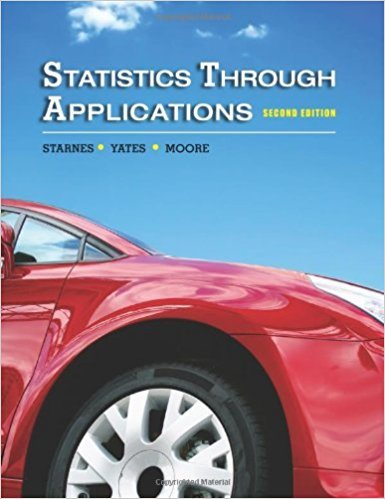×
×

# Solutions for Chapter 10: Inference in Practice## Full solutions for Statistics Through Applications | 2nd Edition

ISBN: 9781429219747Solutions for Chapter 10: Inference in Practice

Solutions for Chapter 10
4 5 0 370 Reviews
22
3
##### ISBN: 9781429219747

Since 68 problems in chapter 10: Inference in Practice have been answered, more than 14556 students have viewed full step-by-step solutions from this chapter. Statistics Through Applications was written by and is associated to the ISBN: 9781429219747. Chapter 10: Inference in Practice includes 68 full step-by-step solutions. This textbook survival guide was created for the textbook: Statistics Through Applications, edition: 2. This expansive textbook survival guide covers the following chapters and their solutions.

Key Statistics Terms and definitions covered in this textbook
• 2 k factorial experiment.

A full factorial experiment with k factors and all factors tested at only two levels (settings) each.

• a-error (or a-risk)

In hypothesis testing, an error incurred by failing to reject a null hypothesis when it is actually false (also called a type II error).

A formula used to determine the probability of the union of two (or more) events from the probabilities of the events and their intersection(s).

• Analysis of variance (ANOVA)

A method of decomposing the total variability in a set of observations, as measured by the sum of the squares of these observations from their average, into component sums of squares that are associated with speciic deined sources of variation

• Average

See Arithmetic mean.

• Average run length, or ARL

The average number of samples taken in a process monitoring or inspection scheme until the scheme signals that the process is operating at a level different from the level in which it began.

• Continuity correction.

A correction factor used to improve the approximation to binomial probabilities from a normal distribution.

• Continuous uniform random variable

A continuous random variable with range of a inite interval and a constant probability density function.

• Control chart

A graphical display used to monitor a process. It usually consists of a horizontal center line corresponding to the in-control value of the parameter that is being monitored and lower and upper control limits. The control limits are determined by statistical criteria and are not arbitrary, nor are they related to speciication limits. If sample points fall within the control limits, the process is said to be in-control, or free from assignable causes. Points beyond the control limits indicate an out-of-control process; that is, assignable causes are likely present. This signals the need to ind and remove the assignable causes.

• Crossed factors

Another name for factors that are arranged in a factorial experiment.

• Cumulative distribution function

For a random variable X, the function of X deined as PX x ( ) ? that is used to specify the probability distribution.

• Cumulative normal distribution function

The cumulative distribution of the standard normal distribution, often denoted as ?( ) x and tabulated in Appendix Table II.

• Defects-per-unit control chart

See U chart

• Empirical model

A model to relate a response to one or more regressors or factors that is developed from data obtained from the system.

• Error variance

The variance of an error term or component in a model.

• Estimate (or point estimate)

The numerical value of a point estimator.

• Expected value

The expected value of a random variable X is its long-term average or mean value. In the continuous case, the expected value of X is E X xf x dx ( ) = ?? ( ) ? ? where f ( ) x is the density function of the random variable X.

• False alarm

A signal from a control chart when no assignable causes are present

• Finite population correction factor

A term in the formula for the variance of a hypergeometric random variable.

• Generator

Effects in a fractional factorial experiment that are used to construct the experimental tests used in the experiment. The generators also deine the aliases.

×